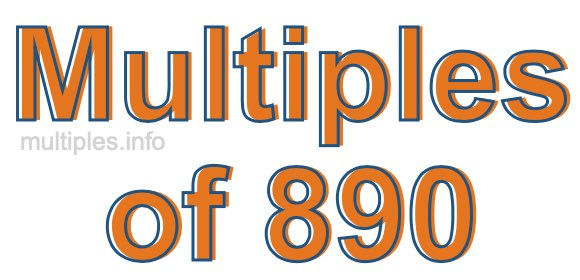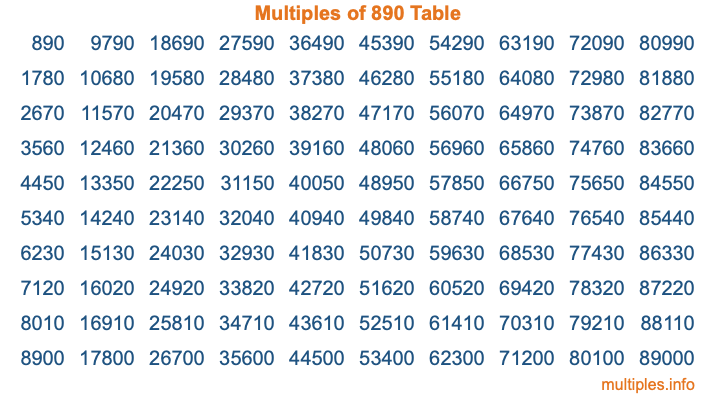Multiples of 890Welcome to the Multiples of 890 page. Here we will first teach you everything you will ever need to know about the multiples of 890, and then give you a study guide summary of everything we taught you to make sure you remember it all. Use this page to look up facts and learn information about the multiples of 890. This page will make you a multiples of eight hundred ninety expert!

Definition of Multiples of 890
Multiples of 890 are all the numbers that when divided by 890 equal an integer. Each of the multiples of 890 are called a multiple. A multiple of 890 is created by multiplying 890 by an integer.

Therefore, to create a list of multiples of 890, you start with 1 multiplied by 890, then 2 multiplied by 890, then 3 multiplied by 890, and so on for as long as you want. Thus, the list of the first five multiples of 890 is 890, 1780, 2670, 3560, and 4450. To see a larger list of multiples of 890, see the printable image of Multiples of 890 further down on this page. We also have a category where you can choose any nth multiple of 890.

Multiples of 890 Checker
The Multiples of 890 Checker below checks to see if any number of your choice is a multiple of 890. In other words, it checks to see if there is any number (integer) that when multiplied by 890 will equal your number. To do that, we divide your number by 890. If the the quotient is an integer, then your number is a multiple of 890.

Is  a multiple of 890?

Least Common Multiple of 890 and ...
A Least Common Multiple (LCM) is the lowest multiple that two or more numbers have in common. This is also called the smallest common multiple or lowest common multiple and is useful to know when you are adding our subtracting fractions. Enter one or more numbers below (890 is already entered) to find the LCM.

Check out our LCM Calculator if you need more details about the Least Common Multiple or if you need the LCM for different numbers for adding and subtraction fractions.

nth Multiple of 890
As we stated above, 890 is the first multiple of 890, 1780 is the second multiple of 890, 2670 is the third multiple of 890, and so on. Enter a number below to find the nth multiple of 890.

th multiple of 890

Multiples of 890 vs Factors of 890
890 is a multiple of 890 and a factor of 890, but that is where the similarities end. All postive multiples of 890 are 890 or greater than 890. All positive factors of 890 are 890 or less than 890.

Below is the beginning list of multiples of 890 and the factors of 890 so you can compare:

Multiples of 890: 890, 1780, 2670, 3560, 4450, etc.

Factors of 890: 1, 2, 5, 10, 89, 178, 445, 890

As you can see, the multiples of 890 are all the numbers that you can divide by 890 to get a whole number. The factors of 890, on the other hand, are all the whole numbers that you can multiply by another whole number to get 890.

It's also interesting to note that if a number (x) is a factor of 890, then 890 will also be a multiple of that number (x).

Multiples of 890 vs Divisors of 890
The divisors of 890 are all the integers that 890 can be divided by evenly. Below is a list of the divisors of 890.

Divisors of 890: 1, 2, 5, 10, 89, 178, 445, 890

The interesting thing to note here is that if you take any multiple of 890 and divide it by a divisor of 890, you will see that the quotient is an integer.

Multiples of 890 Table
Below is an image of the first 100 multiples of 890 in a table. The table is in chronological order, column by column. The first column has the first ten multiples of 890, the second column has the next ten multiples of 890, and so on.The Multiples of 890 Table is also referred to as the 890 Times Table or Times Table of 890. You are welcome to print out our table for your studies.

Negative Multiples of 890
Although not often discussed or needed in math, it is worth mentioning that you can make a list of negative multiples of 890 by multiplying 890 by -1, then by -2, then by -3, and so on, to get the following list of negative multiples of 890:

-890, -1780, -2670, -3560, -4450, etc.

Multiples of 890 Summary
Below is a summary of important Multiples of 890 facts that we have discussed on this page. To retain the knowledge on this page, we recommend that you read through the summary and explain to yourself or a study partner why they hold true.

There are an infinite number of multiples of 890.

A multiple of 890 divided by 890 will equal a whole number.

890 divided by a factor of 890 equals a divisor of 890.

The nth multiple of 890 is n times 890.

The largest factor of 890 is equal to the first positive multiple of 890.

890 is a multiple of every factor of 890.

890 is a multiple of 890.

A multiple of 890 divided by a divisor of 890 equals an integer.

890 divided by a divisor of 890 equals a factor of 890.

Any integer times 890 will equal a multiple of 890.

Multiples of a Number
Here you can get the multiples of another number, all with the same attention to detail as we did for multiples of 890 on this page.

Multiples of
Multiples of 891
Did you find our page about multiples of eight hundred ninety educational? Do you want more knowledge? Check out the multiples of the next number on our list!